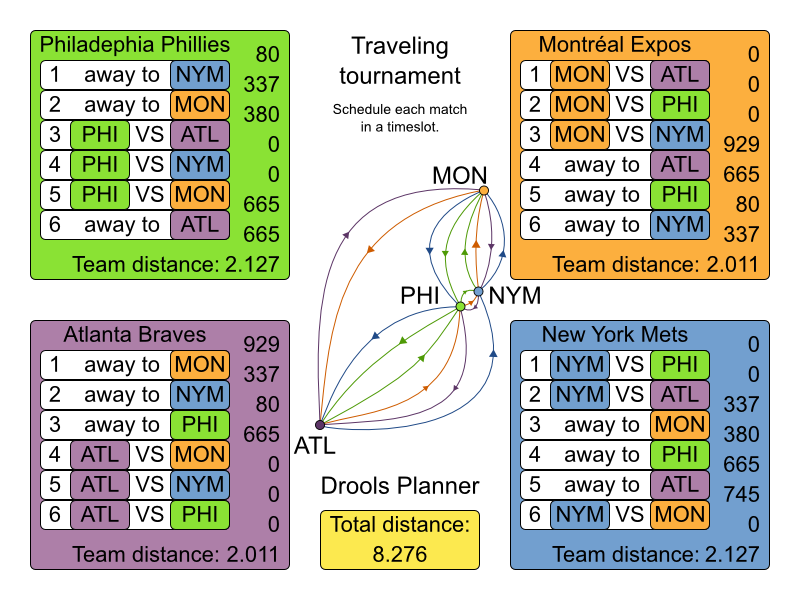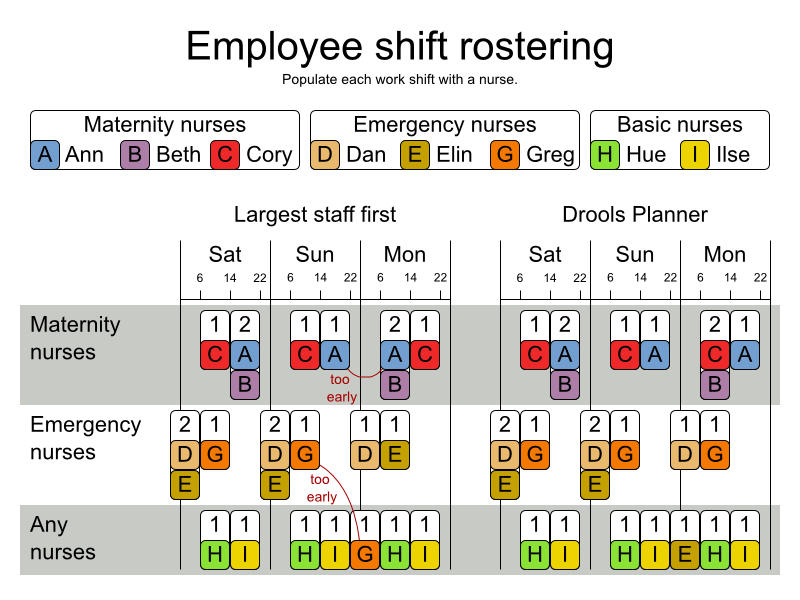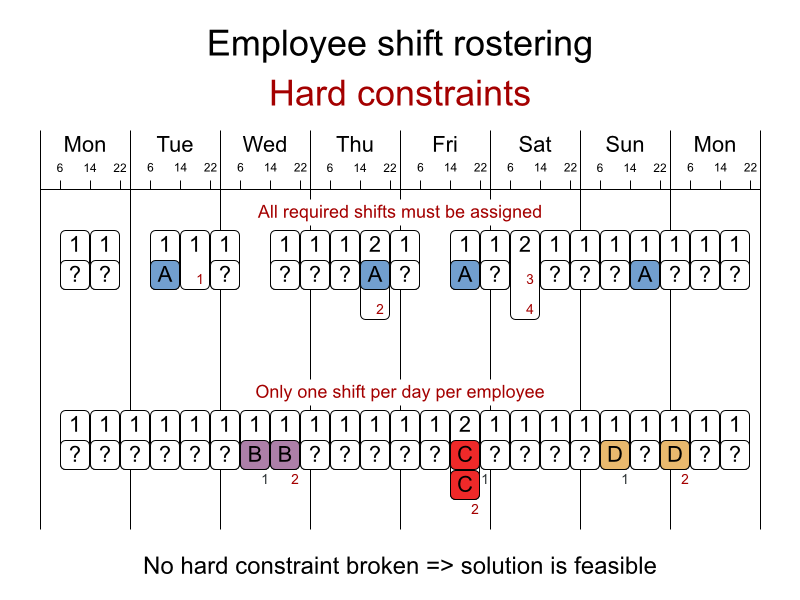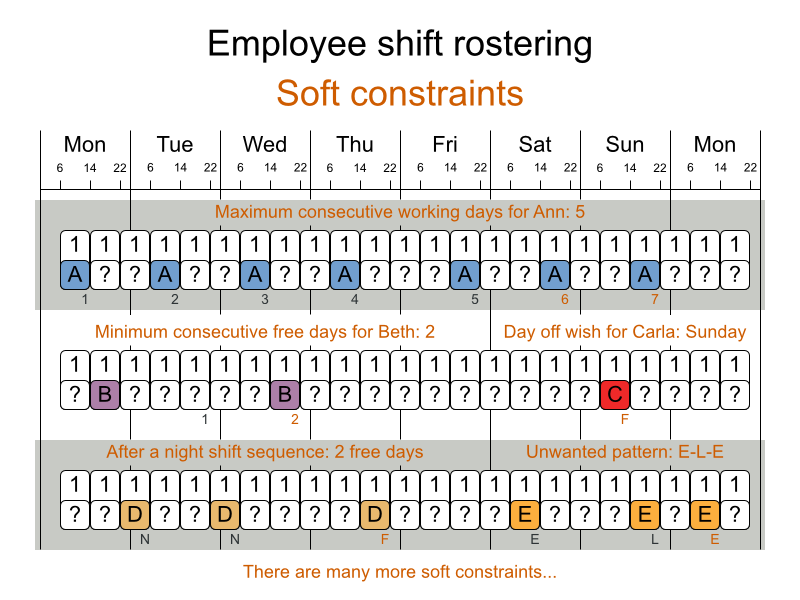## Chapter 2. Use cases and examples

2.1. Introduction
2.2. The n queens example
2.2.1. Problem statement
2.2.2. Solution(s)
2.2.3. Screenshot
2.2.4. Problem size
2.2.5. Domain class diagram
2.3. The Manners 2009 example
2.3.1. Problem statement
2.4. The Traveling Salesman Problem (TSP) example
2.4.1. Problem statement
2.5. The Traveling Tournament Problem (TTP) example
2.5.1. Problem statement
2.5.2. Simple and smart implementation
2.5.3. Problem size
2.6. Cloud balancing
2.6.1. Problem statement
2.7. The ITC 2007 curriculum course example
2.7.1. Problem statement
2.8. The ITC 2007 examination example
2.8.1. Problem statement
2.8.2. Problem size
2.8.3. Domain class diagram
2.9. The patient admission scheduling (PAS) example (hospital bed planning)
2.9.1. Problem statement
2.10. The INRC 2010 nurse rostering example
2.10.1. Problem statement

## 2.2. The n queens example

### 2.2.5. Domain class diagram

Use a good domain model: it will be easier to understand and solve your planning problem with Drools Planner. This is the domain model for the n queens example:

```public class Column {

private int index;

// ... getters and setters
}```
```public class Row {

private int index;

// ... getters and setters
}```
```public class Queen {

private Column column;
private Row row;

public int getAscendingDiagonalIndex() {...}
public int getDescendingDiagonalIndex() {...}

// ... getters and setters
}```
```public class NQueens implements Solution<SimpleScore> {

private int n;
private List<Column> columnList;
private List<Row> rowList;

private List<Queen> queenList;

private SimpleScore score;

// ... getters and setters
}```

A `Queen` instance has a `Column` (for example: 0 is column A, 1 is column B, ...) and a `Row` (its row, for example: 0 is row 0, 1 is row 1, ...). Based on the column and the row, the ascending diagonal line as well as the descending diagonal line can be calculated. The column and row indexes start from the upper left corner of the chessboard.

When 2 queens share the same column, row or diagonal line, such as (*) and (**), they can attack each other.

A single `NQueens` instance contains a list of all `Queen` instances. It is the `Solution` implementation which will be supplied to, solved by and retrieved from the Solver. Notice that in the 4 queens example, NQueens's `getN()` method will always return 4.

## 2.4. The Traveling Salesman Problem (TSP) example

### 2.4.1. Problem statement

It is one of the most intensively studied problems in computational mathematics. Yet, in the real world, it's often only part of a planning problem, along with other constraints, such as employee shift time constraints.

## 2.5. The Traveling Tournament Problem (TTP) example

### 2.5.1. Problem statement

Schedule matches between N teams with the following hard constraints:

and the following soft constraint:### 2.5.2. Simple and smart implementation

There are 2 implementations (simple and smart) to demonstrate the importance of some performance tips. The `DroolsPlannerExamplesApp` always runs the smart implementation, but with these commands you can compare the 2 implementations yourself:

```\$ mvn exec:exec -Dexec.mainClass="org.drools.planner.examples.travelingtournament.app.simple.SimpleTravelingTournamentApp"
...
\$ mvn exec:exec -Dexec.mainClass="org.drools.planner.examples.travelingtournament.app.smart.SmartTravelingTournamentApp"
...```

The smart implementation performs and scales exponentially better than the simple implementation.

## 2.7. The ITC 2007 curriculum course example

### 2.7.1. Problem statement

Schedule lectures into rooms and time periods.

## 2.10. The INRC 2010 nurse rostering example

### 2.10.1. Problem statement

Schedule nurses into shifts.